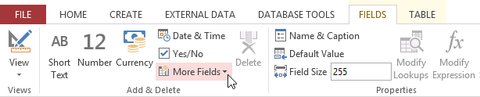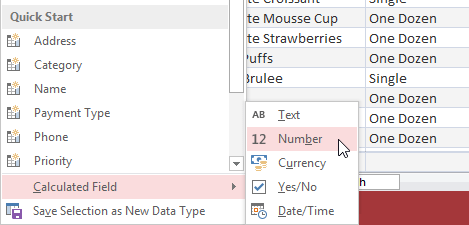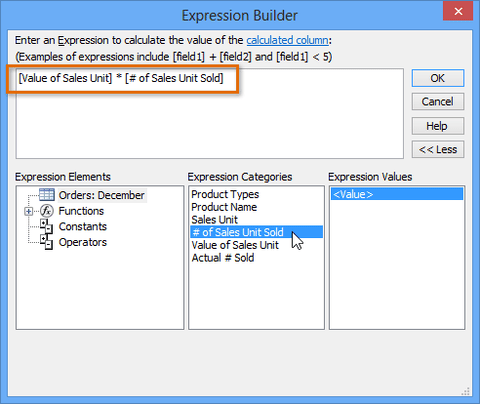# How to insert a calculated field in ExcelYou may not ever need this but if want to know Calculated fields let you perform calculations with the data in your tables. Calculated fields perform calculations using data within one record.

When you create a calculated field, add a field in which every row contains a calculation involving other numerical fields in that row. To do this, you must enter a mathematical expression, which is made up of field names in your table and mathematical symbols. For instance, you could:

• Use + to find the sum of the contents of two fields or to add a constant value (such as + 2 or + 5) to a field
• Use * to multiply the contents of two fields or to multiply fields by a constant value
• Use - to subtract one field from another or to subtract a constant value from a field

Their way to set these calculation fields is to use the Fields tab to access the More Fields menu like this:select the type of data you need:Followed by the mathematical expression (if you are new to this best to use the expression builder):Which give you something like this: